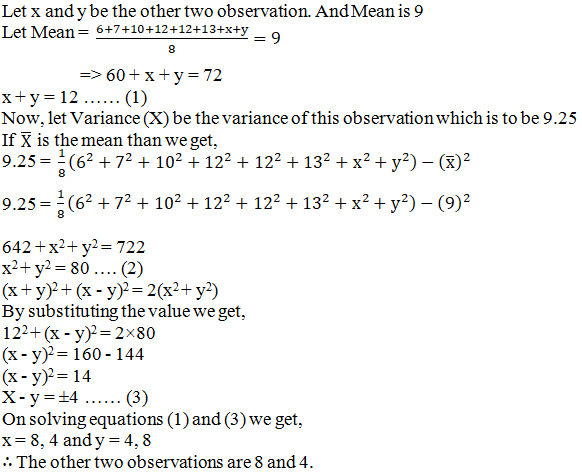# RD Sharma Solutions for Class 11 Chapter 32 - Statistics Exercise 32.4

Exercise 32.4, deals with limitations of mean deviation, variance and standard deviation. Experts at BYJU’S have prepared the solutions in a step by step manner to help students understand the method of solving the problems. To excel in the board examinations, these solutions will boost the confidence level among students, as the concepts are clearly explained and well structured. Students can refer to RD Sharma Class 11 Maths Solutions and download the pdf easily, from the below mentioned links.

## Download the pdf of RD Sharma Solutions for Class 11 Maths Exercise 32.4 Chapter 32 – Statistics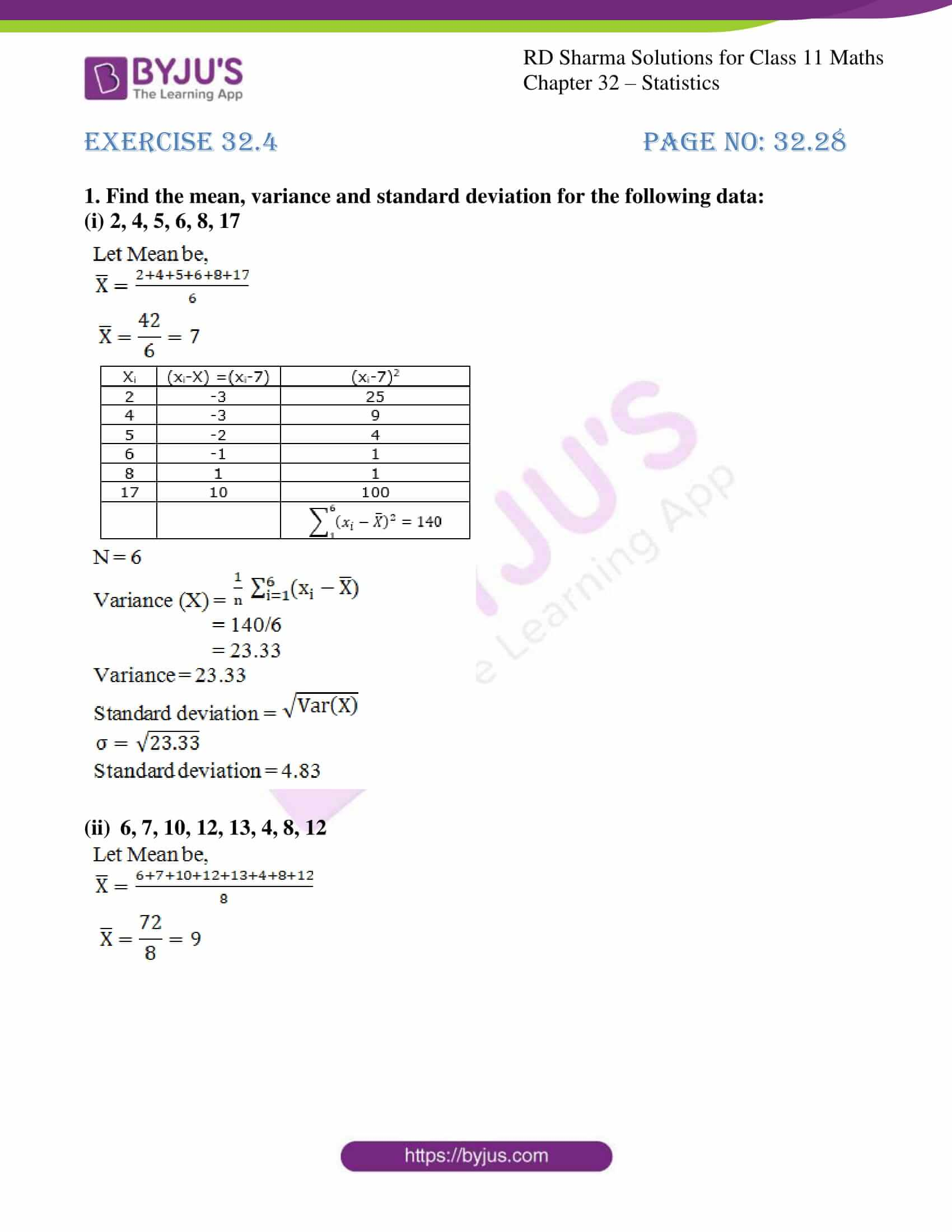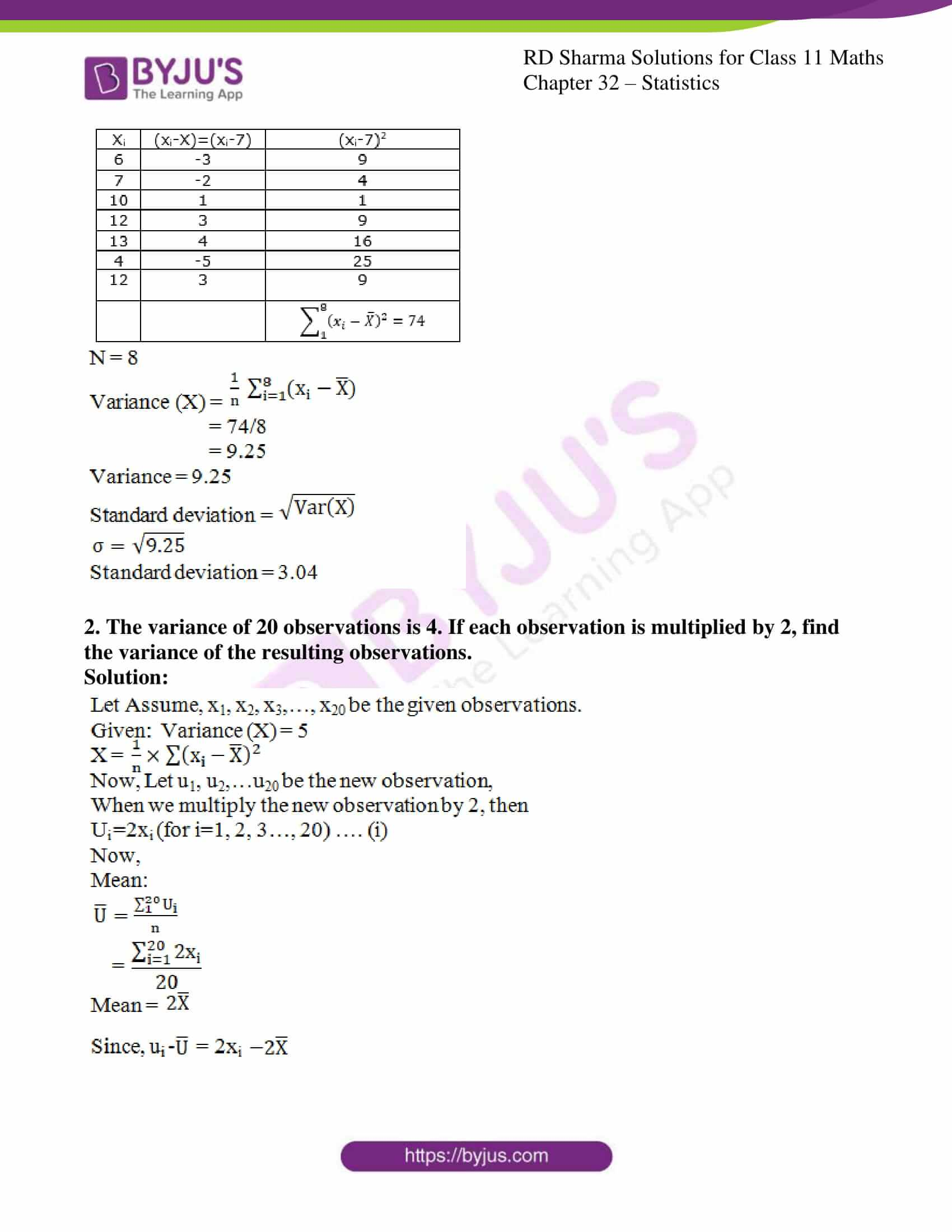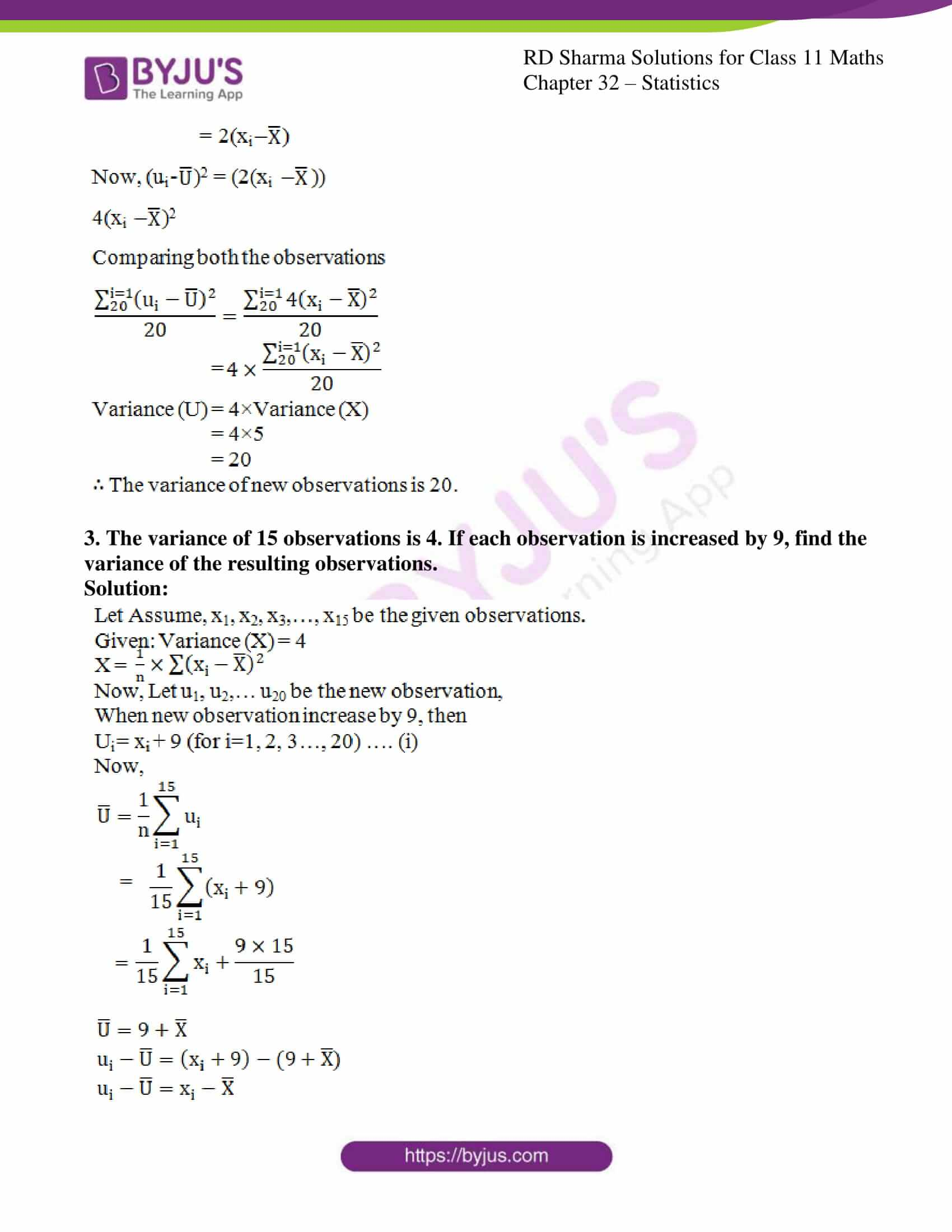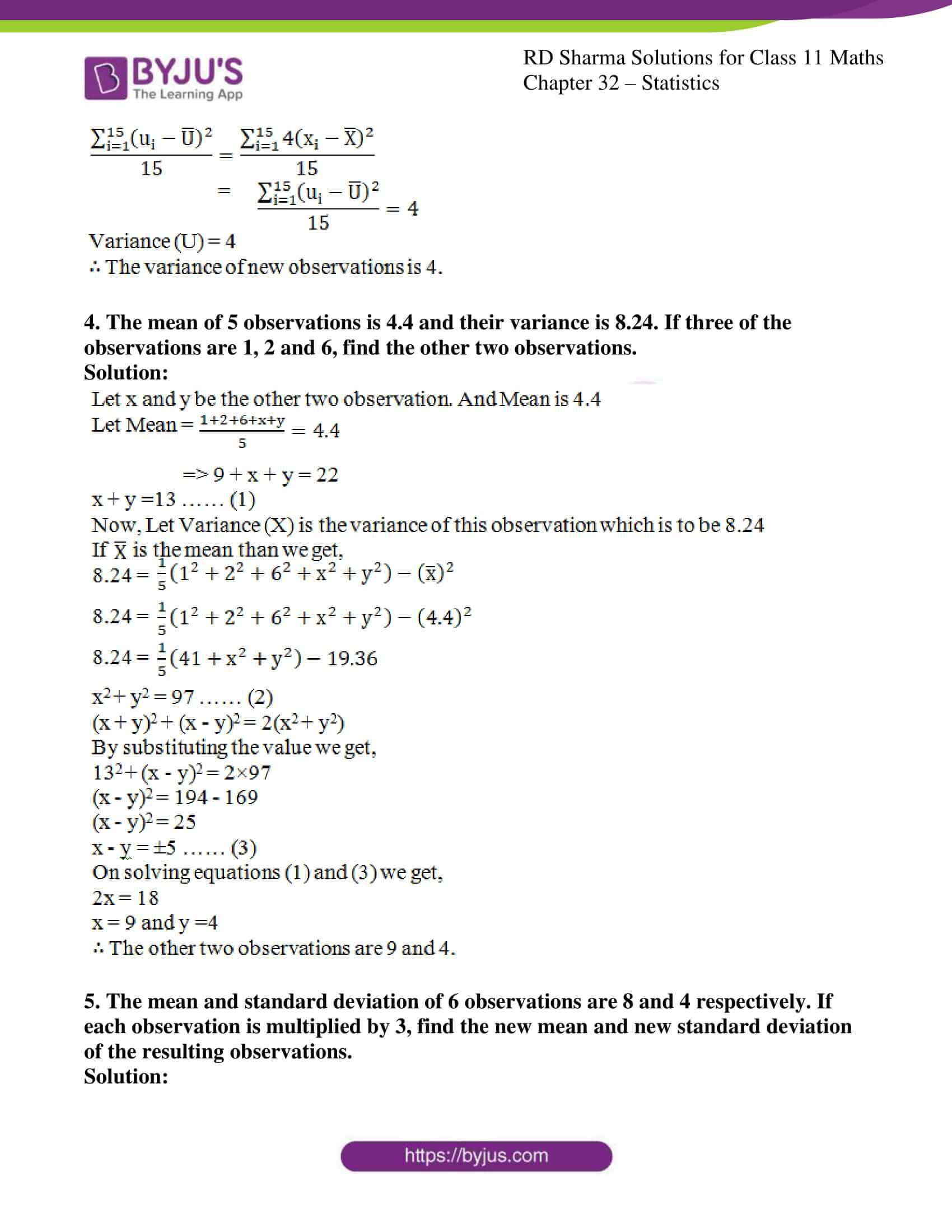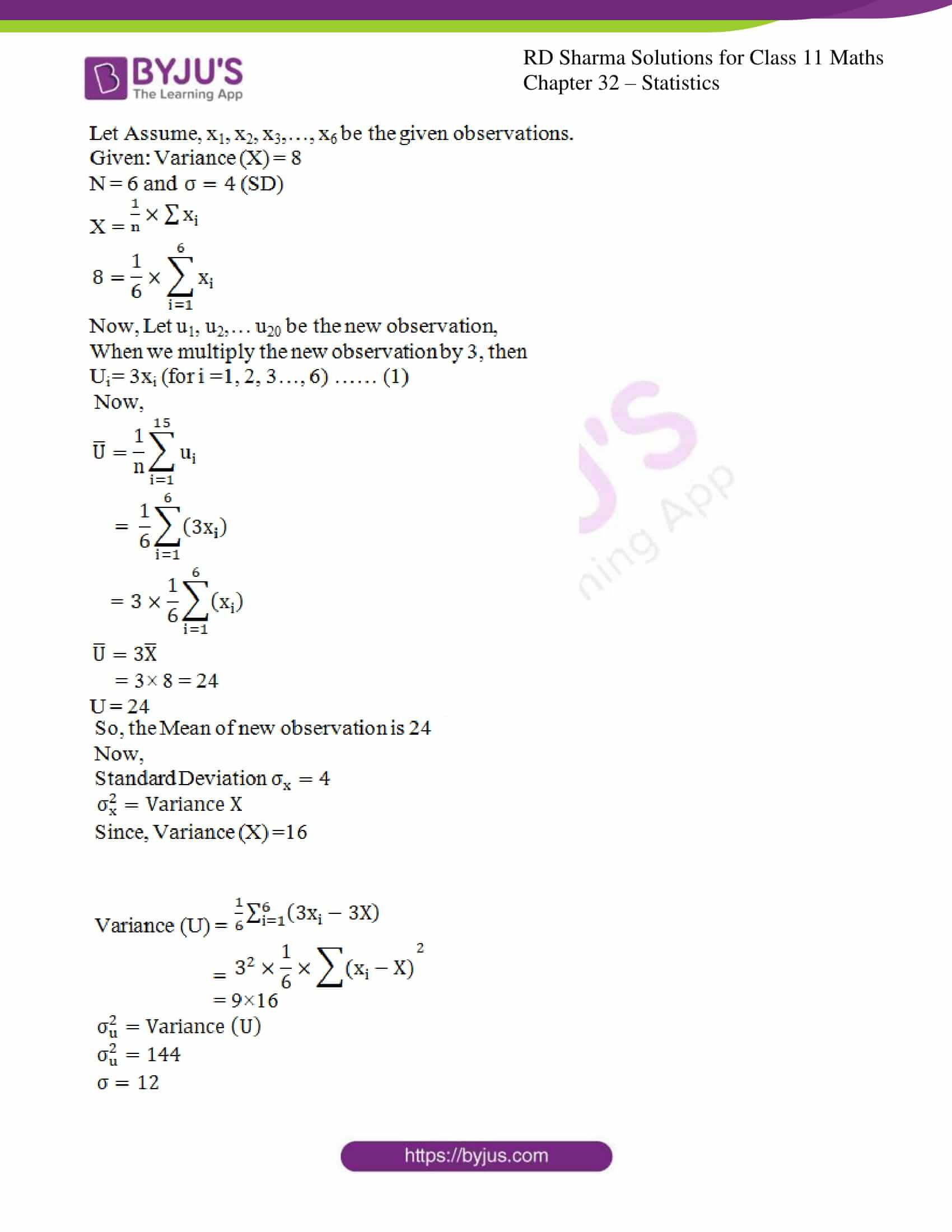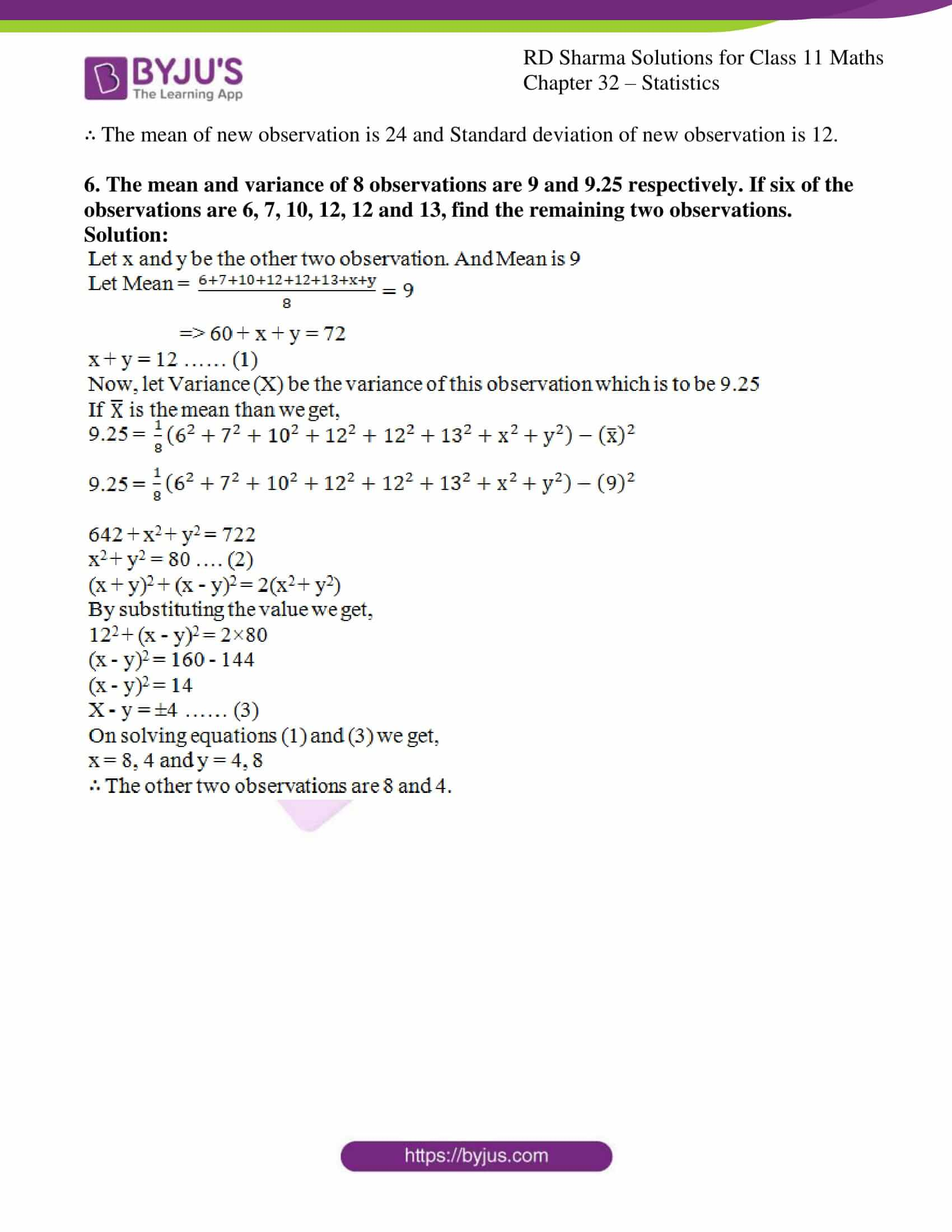### Access answers to RD Sharma Solutions for Class 11 Maths Exercise 32.4 Chapter 32 – Statistics

#### EXERCISE 32.4 PAGE NO: 32.28

1. Find the mean, variance and standard deviation for the following data:
(i) 2, 4, 5, 6, 8, 17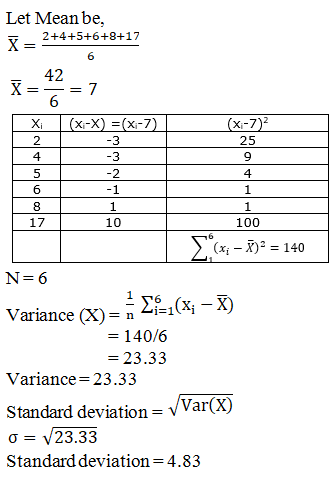(ii) 6, 7, 10, 12, 13, 4, 8, 12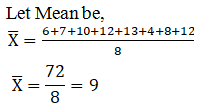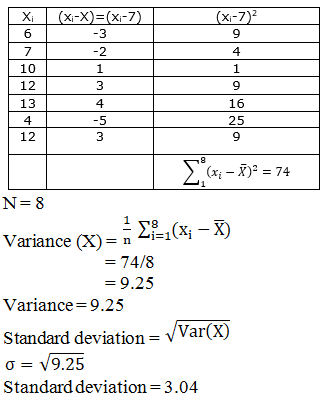2. The variance of 20 observations is 4. If each observation is multiplied by 2, find the variance of the resulting observations.

Solution: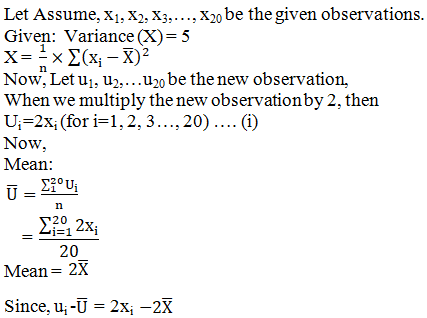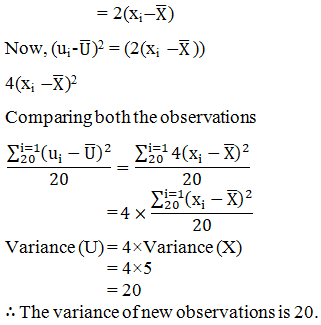3. The variance of 15 observations is 4. If each observation is increased by 9, find the variance of the resulting observations.

Solution: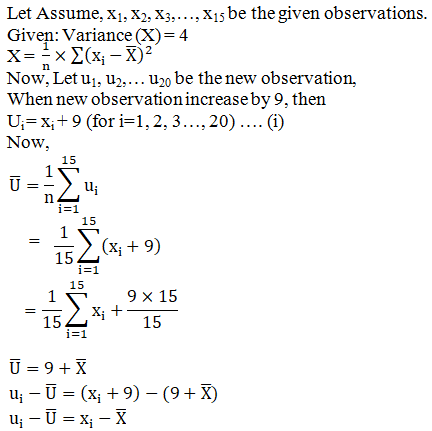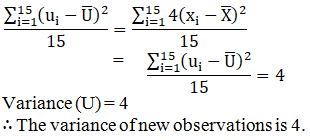4. The mean of 5 observations is 4.4 and their variance is 8.24. If three of the observations are 1, 2 and 6, find the other two observations.

Solution: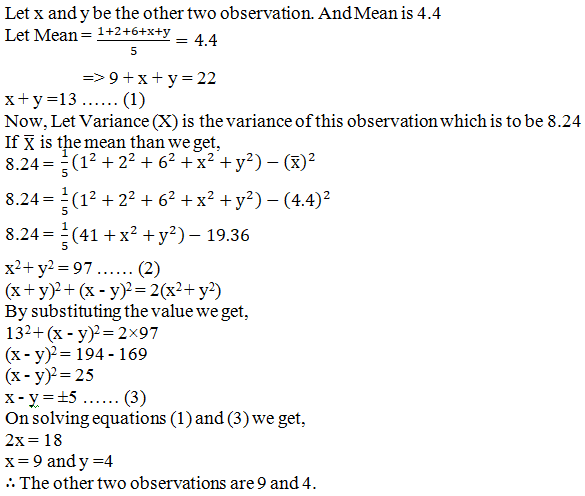5. The mean and standard deviation of 6 observations are 8 and 4 respectively. If each observation is multiplied by 3, find the new mean and new standard deviation of the resulting observations.

Solution: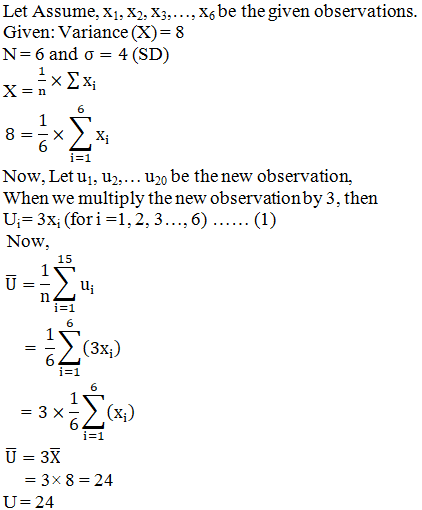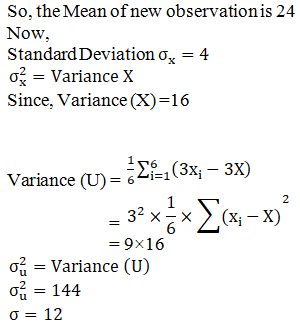∴ The mean of new observation is 24 and Standard deviation of new observation is 12.

6. The mean and variance of 8 observations are 9 and 9.25 respectively. If six of the observations are 6, 7, 10, 12, 12 and 13, find the remaining two observations.

Solution: